# What is a wavenumber (spatial order or space order)?

## Definition

A wavenumber describes the spatial frequency of a periodic quantity (permeance, magnetomotive force, flux density, magnetic force, magnetic vibration), generally along the airgap of the electrical machine or along stator teeth as circumferential wavenumber.

A circumferential wavenumberis the caracteristic frequency of the Fourier harmonic expansion along the airgap of quantity: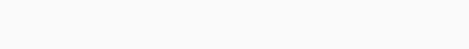The period of the wave isand the wave length is. The wavenumberalso corresponds to the number of maxima and number of minima of the wave along the airgap (half the number of nodes). As an example, due to the fact that magnetic forces are proportional to the square of the flux density, the highest magnitude circumferential wavenumber of magnetic forces is given by.

The term space order should be avoided or used to define the multiple between number of pole pairs p and wavenumber. For instance the space order of wavenumberequals 2.

When extending the Fourier expansion to time, one obtains for a single frequency wave:

(1)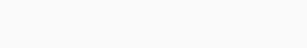The mechanical velocity (or phase group velocity, which is also the travelling speed of the wave nodes) is given by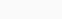. The direction of the travelling wave depends on the sign of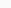and: in MANATEE software, frequencies are kept positive but wavenumbers can be negative to account for opposite travelling direction.

This means that a vibration wave of wavenumberand electrical frequency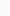has a mechanical velocity given by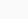– it is the velocity of its nodes.

## Animations and illustrations

Some animations of single frequency travelling waves of wavenumber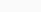toare given below.

Circumferential wavenumberis called pulsating or pulsing, it has no nodes. Circumferential wavenumber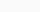of harmonic forces is called Unbalanced Magnetic Pull.

When carrying an FFT on lumped magnetic forces as in Manatee software one can find the following force wavenumbers:

The notion of wavenumbers can be extended to caracterize how harmonics evolve along machine axial length with longitudinal wavenumbers, noted s in MANATEE software. As an example, the following animations illustrate a radial Maxwell stress wave (,) and a tangential Maxwell stress wave (,):

In axial flux machines, one can also introduce the notion of radial wavenumbers.

## Application to e-NVH

The use of wavenumbers is very useful when analyzing electromagnetic noise and vibrations of electrical machines because resonances do not only depend on electromagnetic excitation frequency, they also depend on the match between structural modes and magnetic force shape (described by the wavenumber).

The higher the force wavenumber, the lower is the displacement magnitude due to increased stiffness of the yoke (roughly in the power 4 of). The vibration and noise analysis of electrical machines can therefore focus on low wavenumbers (from 0 to 18 depending on the topology and size of the machine). However, due to the quadratic nature of magnetic forces, high flux density wavenumbers can generate low magnetic force wavenumbers. As an example in a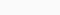stator slot PMSM with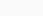pole pairs, the combination of flux density harmonics of wavenumbers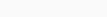and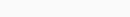give rise to a magnetic force of wavenumber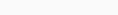.

## Application to MANATEE

MANATEE software helps identifying all flux density and force wavenumbers involved in acoustic noise generation.